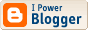# Shantanu's Blog

Database Consultant

## analyse general logs

It is possible to analyze general logs using percona query digest:

apt-get install percona-toolkit_2.2.10_all.deb
mk-query-digest --type genlog  study2.txt

## converting dates in pandas

Sometimes I get the date column from the csv string is year-month-day without any space or hyphen. for e.g. 120814
This can be converted to regular date using a function and that function can be called in the read_csv method.

import pandas as pd
import datetime as dt

def mydate(x):
try:
return dt.datetime.strptime(x, '%y%m%d')
except ValueError:
return pd.NaT

vt_data = [ 'vt_code', 'vt_id' , 'point_date' ,  'deviation' ]

vts = pd.read_csv('test.txt', sep=',', names=vt_data, parse_dates=, date_parser=mydate)
Another option is not to do parsing as you read the data in, then set the index as shown below.

df.index = pd.to_datetime(df["ticketDate"] + df["ticketTime"], coerce=True, format='%Y-%m-%d %H:%M:%S')

The coerce=True forces bad values to NaT

Labels: ,

## converting datetime

Usually you need to call strptime or use dateutil to parse date strings. However in this case, rawtime looks similar enough to a date as specified by the RFC 2822 (Internet Message Format) that it can parsed using the standard library function :
`import email.utils as EU `
`import datetime as DT `
`rawtime = "Wed Jun 25 15:38:29 PDT 2014" `
`timetup = EU.parsedate(rawtime) `
`# (2014, 6, 25, 15, 38, 29, 0, 1, -1) `
`date = DT.datetime(*timetup[:6]) `
`print(date)  # 2014-06-25 15:38:29  `

To see the article visit http://www.feedspot.com/article/FUHFs2If5+0Z5ukb

## pandas and mysql

You can connect to mysql database using pandas module.

import pymysql
conn = pymysql.connect(host='127.0.0.1', port=3306, user='root', passwd='', db='mysql')

import pandas as pd
pd.read_sql('select Host from user', conn, index_col=None, coerce_float=True, params=None, parse_dates=None, columns=None)

## Archives